Kinematics homework Help at TutorEye

## Kinematics:

Kinematics is the study of motion of the body and its system without the consideration of actual cause of motion. It is independently described by the motion of point, object and system of objects. It is a part of classical mechanics, which deals in the pure motion of the body without any reference of force or external factors causing the motion.

## Kinematics Sample Questions:

Question 1: Which of the following is the correct relation between velocity and acceleration?

a) v.ds = a.dv        b) v.dv = a.ds

c) v.ds = a.dv        d) v.ds = a.ds

Explanation: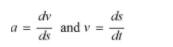Question 2: Find the value of acceleration at 1s from the given expression of velocity with displacement.

a) 208        b) 210

c) 104        d) 205

Explanation: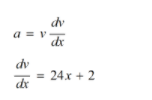Question 3: The expression of angular momentum represented in terms of mass, radius and angular velocity is

(a)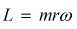(b)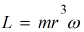(c)(d)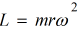Answer:  (c)Explanation: Since, angular momentum

Question 4:  A body is travelling with initial velocity 5m/s and acceleration 2m/s². Find the time when the velocity of the body becomes 50m/s.

a) 20 s          b) 22 s

c) 12 s          d) 22.5 s

Explanation: Given velocities

Question 5: A body moving with the velocity of 5m/s  decelerates at rate dv/dt = - 2√v. The time taken by the body to come to rest.

a) 2 s                 b) 2.24 s

c) 2.42 s            c) 2.1 s

Explanation: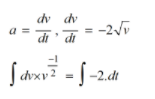Question 6: A body travelling in a straight line is represented by the following equation. The initial velocity and displacement are respectively. The value of constant k is

a)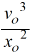b)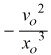c)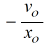d)Answer:  d)Explanation:Question 7: In a pure rolling motion, the velocity at point of contact is

a) non-zero                     b) zero

c) greater than zero        d) none of the above

Explanation: In a pure rolling motion, the relative velocity at point of contact is always zero

Question 8: A circular plane of radius 5m is rotating with a velocity of 50m/s. Calculate the velocity of particles revolving along the plane at a distance of 4m from the center?

a) 20 m/s          b) 30 m/s

c) 10 m/s          d) 40 m/s

Explanation: Given,

Question 9: A body of mass 2 kg, initially at rest is acted by time varying force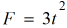. Find the distance travelled by the mass in 2 s.

a) 2 m          b) 1 m

c) 4 m          d) 3 m

Explanation:  Applying Newton’s 2nd law,

Question 10: In a circular motion, the tangential and radial acceleration tells the change in ______________ respectively.

a) Magnitude, speed          b) Displacement, velocity

c) Velocity, speed              d) Magnitude, direction